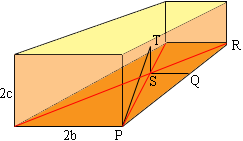SEARCH HOMEMath Central Quandaries & QueriesQuestion from Diana, a student: Consider a rectangular room, 15 feet wide, 30 feet long and 12 feet high. What is the exact distance from any of the 8 corners of the room and its geometric center? Can you write a generic formula for such a distance? And if we keep the same proportions on all dimensions, can you write an expression for the same distance as a function of the floor perimeter?Hi Diana,

I can help you write a generic formula. I let the rectangular room have dimensions 2a feet by 2b feet by 2c feet.Q is the midpoint of PR so the distance from P to Q is a feet. Triangle QSP is a right triangle so use Pythagoras theorem to find the length of SP.

T is the midpoint of the vertical line segment from S to the ceiling of the room. Triangle STP is a right triangle so use Pythagoras again, this time to find the length of TP.

I hope this helps,
PennyMath Central is supported by the University of Regina and The Pacific Institute for the Mathematical Sciences.